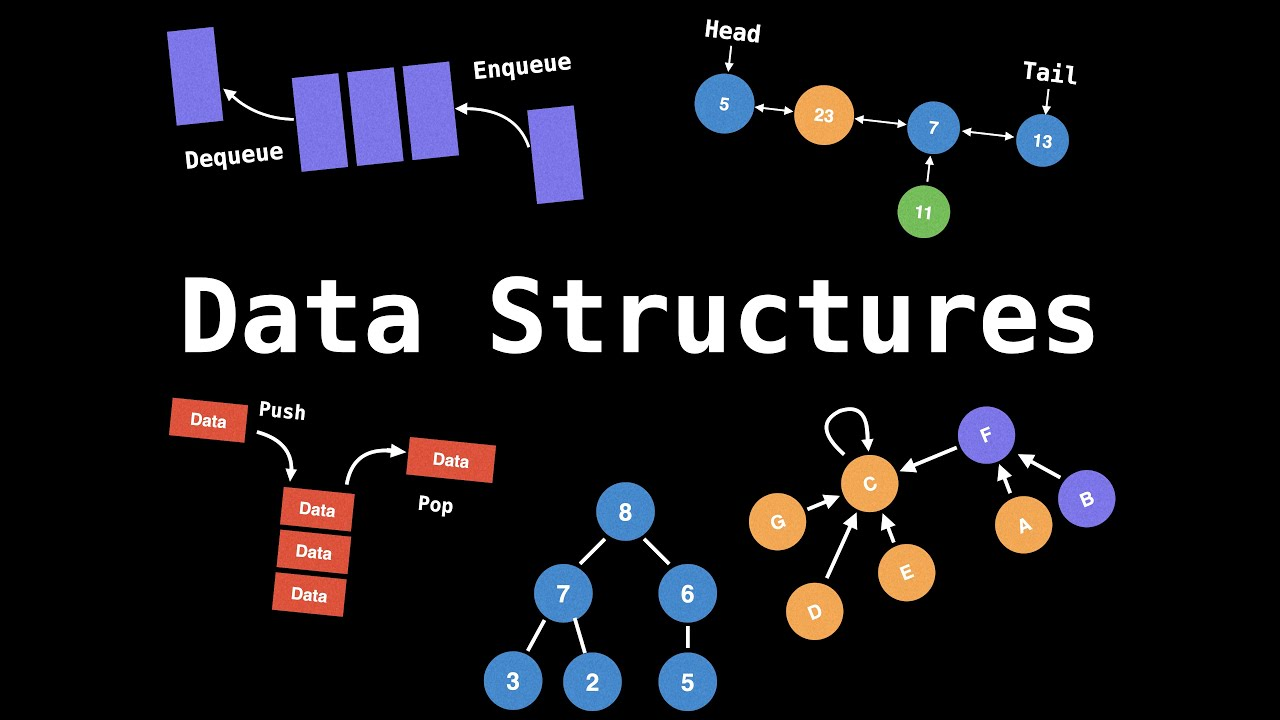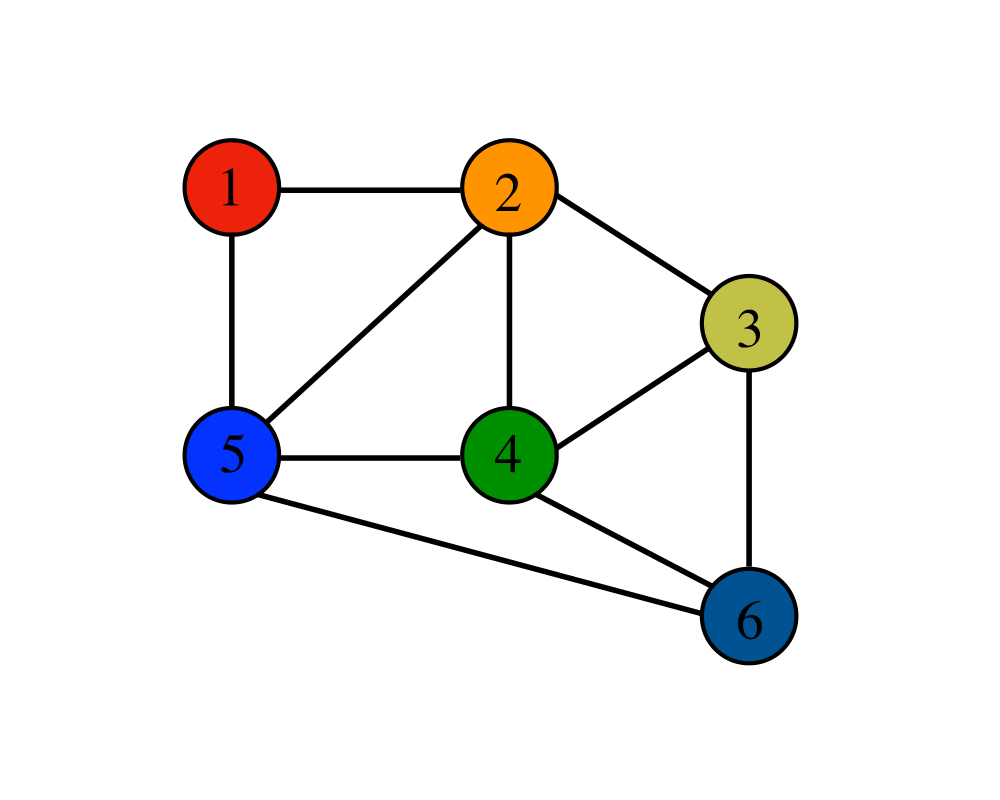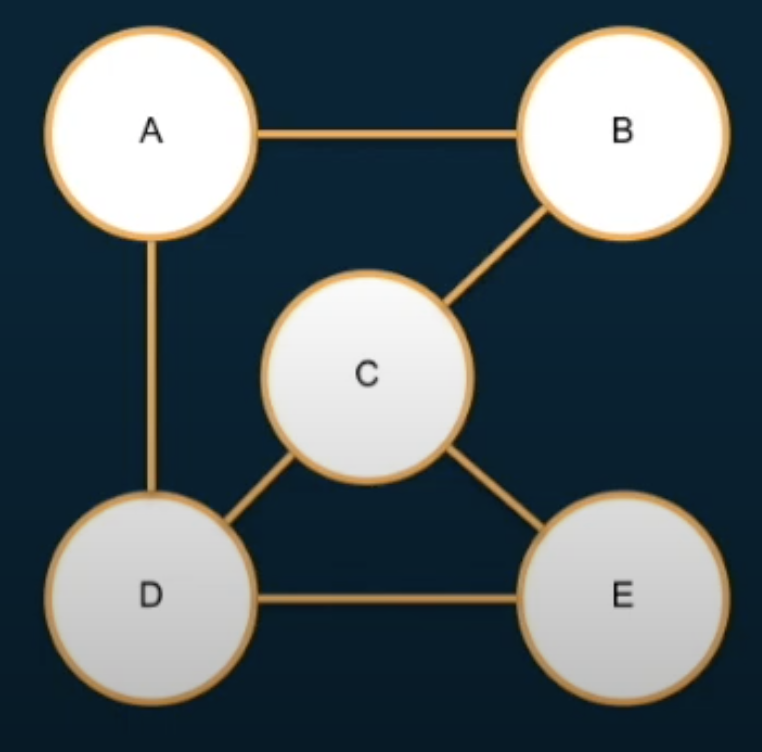# 8. Graph Data Structure

kimkevin90·2021년 11월 29일
0## 1. What is a graph?• A data structure made up of nodes or vertices and edges or the connections between nodes

• Typically, a visualization of a graph will be of nodes represented by circles and edges as lines between the circles

Trees vs Graph

• Trees art just a special kind of graph with one root and only on unique path between any two nodes

• A graph can go beyond that and have any number of root elements and multiple paths between nodes

## 2. How can we represent a graph in code?1) Vertex list + Egde list

const vertices = ['A', 'B', 'C', 'D', 'E'];

const edges = [
['A', 'B'],
['A', 'D'],
['B', 'C'],
['C', 'D'],
['C', 'E'],
['D', 'E']
]

const findAdjacentNodes = (node) => {

// Loop through edges array
// Is my node in the connection?
// If yes, push the other node in pair, into adjacentNodes array
// If no, keep looping

for (let edge of edges) {
const nodeIdx = edge.indexOf(node);
if (nodeIdx > -1) {
let adjacentNode = nodeIdx === 0 ? edge : edge
}
}

};

const isConnected = (node1, node2) => {
return edges.some((edge) => {
return edge.indexOf(node1) > -1 && edge.indexOf(node2) > -1
})
};

2) Ajacency Matrix

• A 2-D array filled out with 1's and O's where each array represents a node and each index in the subarray, represents a potentail connection to another node
• The value at adjacencyMatrix[node1][node2] indicates where there is a connection between node1 and node2
const vertices = ['A', 'B', 'C', 'D', 'E'];

const vertexIdxs = {
'A': 0,
'B': 1,
'C': 2,
'D': 3,
'E': 4,
};

[0,1,0,1,0],
[1,0,1,0,0],
[0,1,0,1,1],
[1,0,1,0,1],
[0,0,1,1,0],
];

const findAdjacencies = (node) => {

// get the row in the matrix
// loop through the row
// if there is 1, push that node
// otherwise skip
for (let i = 0; i < vertices.length; i++) {
let nodeVertext = vertexIdxs[node];
}
}

}

// isConnected
const isConnected = (node1, node2) => {
const nodeIdx1 = vertexIdxs[node1];
const nodeIdx2 = vertexIdxs[node2];

};


3) Ajacency List

• For every node, store a list of what nodes it's connecte to
class Node {
constructor(value) {
this.value = value;
this.edgeList = [];
}

connect(node) {
this.edgeList.push(node);
node.edgeList.push(this);
}

return this.edgeList.map(edge => edge.value);
}

isConnect(node) {
return this.edgeList.map(edge => edge.value).indexOf(node.value) > -1;
}
}

class Graph {
constructor(nodes) {
this.nodes = [...nodes];
}

this.nodes.push(node);
}
}

const nodeA = new Node('A');
const nodeB = new Node('B');
const nodeC = new Node('C');
const nodeD = new Node('D');
const nodeE = new Node('E');

const graph = new Graph([nodeA, nodeB, nodeC, nodeD, nodeE]);

nodeA.connect(nodeB);
nodeA.connect(nodeD);
nodeB.connect(nodeC);
nodeC.connect(nodeD);
nodeC.connect(nodeE);
nodeD.connect(nodeE);

// for (let node of graph.nodes) {
//   console.log(Node ${node.value}); // for (let connectedNode of node.edgeList) { // console.log(Node${node.value} is connected to \${connectedNode.value});
//   }
// }

console.log(nodeA.isConnect(nodeB));